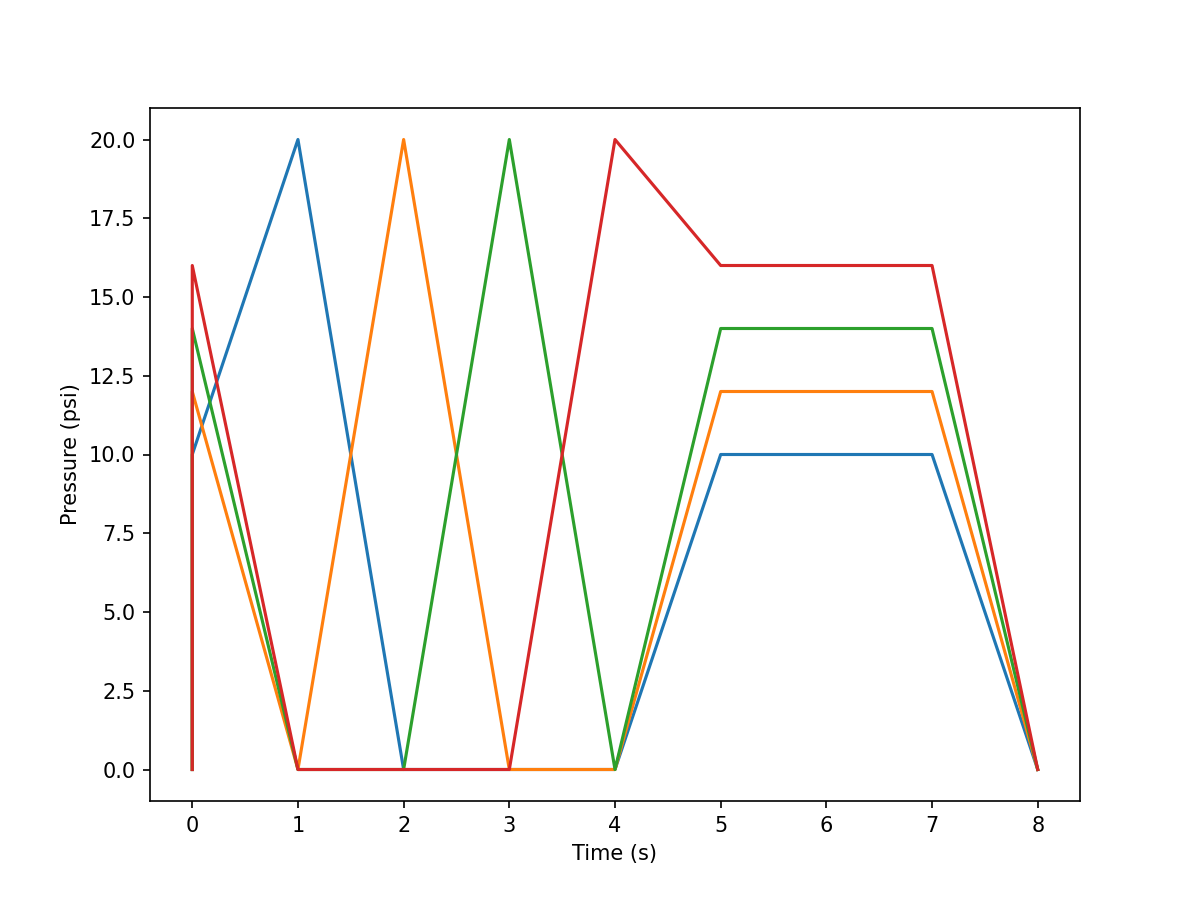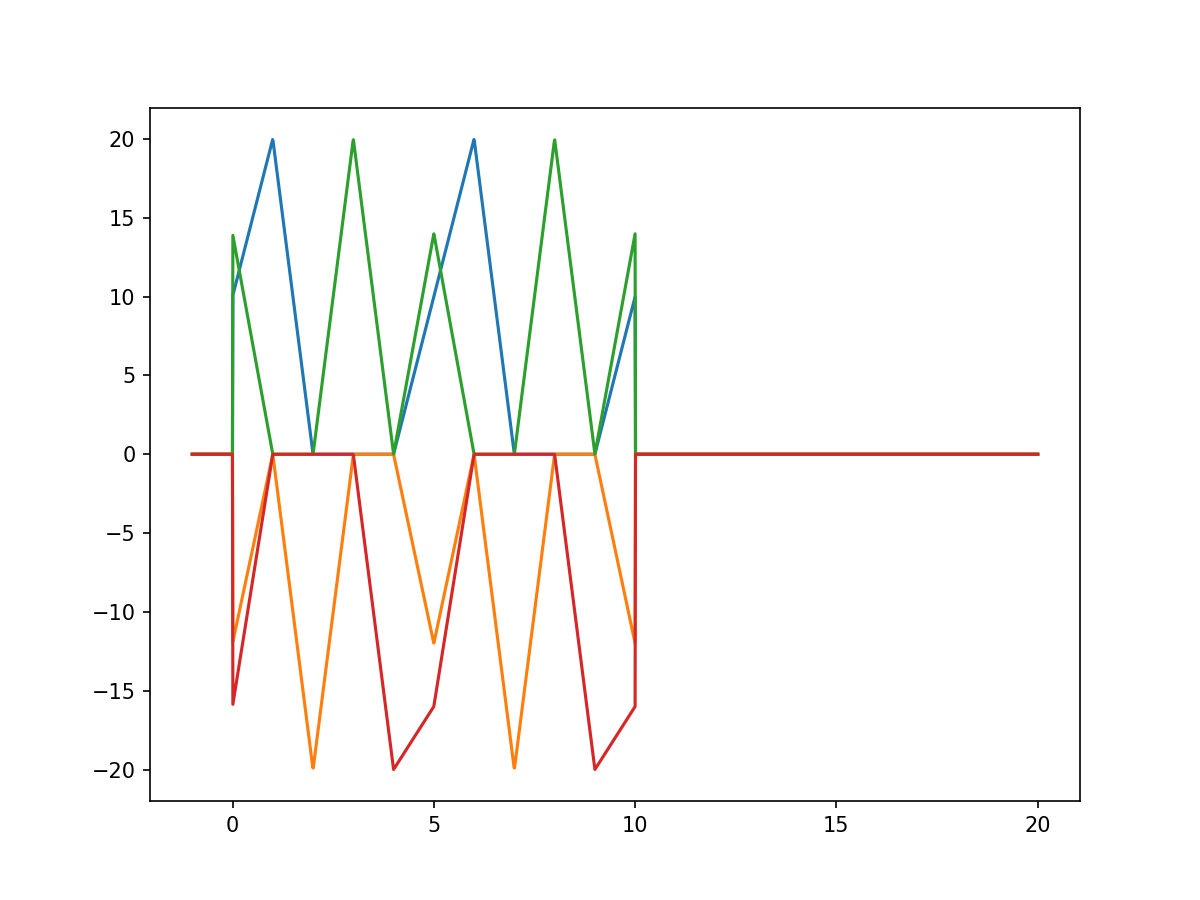# Build One Trajectory

In this example, we will build one trajectory from a definition file, create an interpolator, and use that interpolator to generate a graph.

## Trajectory Definition

Trajectories are defined in YAML files as either a series of waypoints, or simple periodic functions. Here we will look at a typical waypoint trajectory:

```# A simple waypoint trajectory with prefix and suffix

# Top-level settings
settings:
traj_type: 'direct'  # Types include: 'waveform', 'interp', 'direct'

# Place all of the type-specific settings in here
config:
setpoints:
# [time, finger1, finger2, n/c, n/c]
main:
- [0.0,   10, 12, 14,  16]
- [1.0,    20, 0, 0,  0]
- [2.0,   0, 20, 0,  0]
- [3.0,     0, 0, 20, 0]
- [4.0,     0, 0, 0, 20]
- [5.0,    10, 12, 14, 16]

prefix:
- [0.000,   0, 0, 0,  0]
- [1.0,    10, 12, 14,  16]

suffix:
- [2.000,   10, 12, 14,  16]
- [3.0,  0, 0, 0,  0]
```

All trajectories can have three component:

1. `prefix` - Runs once at the beginning of the trajectory

2. `main` - Can be looped over and over (ensure the first and last waypoint are equal)

3. `suffix` - Runs once at the end of the trajectory

Within each trajectory component, each waypoint is defined as a list:

• arg #0 - Time (in seconds)

• args #1-N - Waypoint values for each signal

## Trajectory Builder

We need to build this trajectory before we can use it. To do this, we use a `TrajBuilder` object, and give it a file to load the definition from.

```file_to_use = 'traj_setup/setpoint_traj_demo.yaml'
builder = sorotraj.TrajBuilder() # Make a traj-builder object
traj = builder.get_trajectory()
```

Note

We can also directly load a trajectory definition py passing a dictionary to the `TrajBuilder`: `builder.set_definition(def_dict)`

Now that we have the trajectory (`traj`), let’s plot it:

```builder.plot_traj()
```## Trajectory Interpolator

Now we want to create an interpolator so we can have a convenient way to get trajectory values given arbitrary values of time. We do this with an `Interpolator` object. Here, we want to get an interpolator function that loops the `main` trajectory component 2 times, with a speed factor of 1.0 (times as defined in the definition), and invert signals 1 and 3 (these are indices).

```interp = sorotraj.Interpolator(traj)

# Get the actuation function for the specified run parameters
actuation_fn, final_time = interp.get_traj_function(
num_reps=2,
speed_factor=1.0,
invert_direction=[1,3])
```

Now that we have an interpolation function we can input a time (or vector of times) and obtain the trajectory at arbirary times. This function is useful when performing simulations of soft systems.

In this example, we go from -1 (before the start of the trajectory) to 20 sec. (well beyond the end of the trajectory).

```times = np.linspace(-1,20,2000)
vals = actuation_fn(times)

plt.figure(figsize=(8,6),dpi=150)
plt.plot(times, vals)
plt.show()
```## Full Code

Here’s the whole example in one file:

```import sorotraj
import numpy as np
import matplotlib.pyplot as plt

#file_to_use = 'traj_setup/setpoint_traj_demo.yaml'      # Basic demo
#file_to_use = 'traj_setup/setpoint_traj_demo_err0.yaml' # duplicate time (will throw exception)
#file_to_use = 'traj_setup/setpoint_traj_demo_err1.yaml' # non-monotonic time (will throw exception)
#file_to_use = 'traj_setup/setpoint_traj_demo_0.yaml'    # empty prefix
#file_to_use = 'traj_setup/setpoint_traj_demo_1.yaml'    # single line prefix
file_to_use = 'traj_setup/setpoint_traj_demo_2.yaml'    # No prefix or suffix
#file_to_use = 'traj_setup/waveform_traj_demo.yaml'    # single prefix line

# Build the trajectory from the definition file
builder = sorotraj.TrajBuilder()
traj = builder.get_trajectory()
for key in traj:
print(key)
print(traj[key])

# Plot the trajectory
builder.plot_traj(fig_kwargs={'figsize':(8,6),'dpi':150})

# Make an interpolator from the trajectory
interp = sorotraj.Interpolator(traj)

# Get the actuation function for the specified run parameters
actuation_fn, final_time = interp.get_traj_function(
num_reps=1,
speed_factor=1.0,
invert_direction=False)

print("Final Interpolation Time: %f"%(final_time))

# Get the cycle function for the specified run parameters
cycle_fn = interp.get_cycle_function(
num_reps=1,
speed_factor=1.0,
invert_direction=False)

# Plot the actuation function vs. time
times = np.linspace(-1,10,2000)
vals = actuation_fn(times, debug=True)

plt.figure(figsize=(8,6),dpi=150)
plt.plot(times, vals)
plt.show()

abs_times = builder.get_absolute_times(num_reps=2, speed_factor=1.0)
abs_vals  = actuation_fn(abs_times)

print("Flat Trajectory:")

traj_flat = builder.get_flattened_trajectory(num_reps=2, speed_factor=1.0, invert_direction=[1,3])

print("Times")
print(abs_times)
print("Values")
print(traj_flat)
```